﻿ Research on Topology, Control and Simulation of DVRPublications are Open
Access in this journal
Article Versions
Export Article
• Normal Style
• MLA Style
• APA Style
• Chicago Style
Research Article
Open Access Peer-reviewed

### Research on Topology, Control and Simulation of DVR

Yuqi Pang , Xiaotian Xu, Xunyu Liu, Xinyuan Zhang
American Journal of Electrical and Electronic Engineering. 2020, 8(4), 102-107. DOI: 10.12691/ajeee-8-4-1
Received July 12, 2020; Revised August 14, 2020; Accepted August 23, 2020

### Abstract

Dynamic voltage restorer (DVR) is a series compensation device with energy storage device, which can compensate reactive power and active power. DVR is composed of power module, action module, control module and sampling module. The core of the research lies in its topology and control strategy. This paper starts from the above two aspects, studies the working principle and structural characteristics of the dynamic voltage restorer, and analyzes the topological structure and the function of the structure of the dynamic voltage restorer. A voltage and current double closed-loop control strategy is proposed, which can effectively compensate the transient voltage. A DVR model is built in Matlab/Simulink, and power failure and three-phase short circuit are simulated. A double closed-loop control strategy based on voltage and current is applied. The simulation results show the feasibility and effectiveness of the control strategy.

### 1. Introduction

The main characteristic of voltage sag is the instantaneous drop in voltage. Since the load is always connected to the power supply, the sag is easier to be ignored in the actual power consumption process than the interruption 1, 2, but the voltage sag occurs more frequently. In many cases, voltage sags are a direct threat to sensitive electrical equipment, and may cause industrial shutdowns and ultimately cause major safety accidents. Due to the limited elimination time of the voltage sag caused by the fault and the wide spread from the transmission and distribution system to the low-voltage load, it is almost impossible to avoid the voltage sag problem at present 3. The device can alleviate the sag problem to a greater extent by intelligently controlling the device or installing large capacitors in each device. In real power systems, dynamic voltage restorers (DVRs) are usually installed to reduce voltage sags and minimize the risk of load tripping during sags 4, 5.

Reference 6, 7 introduces that the battery energy storage DVR with adaptive neuron control can compensate for the voltage drop of the microgrid, and has strong dynamic response and anti-disturbance capabilities. Reference 8 proposes a DVR control strategy based on dual-loop vector decoupling control to improve the dynamic performance of the DVR in the compensation process. Reference 9 introduces a cascaded H-bridge dynamic voltage restorer, and proposes a control strategy that automatically adjusts the phase angle of the load reference voltage based on the minimum energy compensation when the DC side voltage is fixed. In reference 10, a control strategy based on multiple proportional resonance is proposed for the fundamental wave voltage extraction and harmonic compensation of dynamic voltage restorer in the single-phase voltage compensation process. Reference 11 studies the new dynamic voltage restorer (DVR) system based on supercapacitor energy storage, which effectively utilizes the extremely high charging-discharge capacity and charge storage capacity of the supercapacitor, so that the dynamic voltage restorer can quickly provide stable voltage when compensating the voltage

It can be seen that the research on DVR mainly focuses on the control mode and topology. This paper studies the topological and the function of the structure of the DVR, and proposes a voltage and current double closed-loop control strategy, which has strong dynamic response ability and anti-disturbance ability. Finally, built an AC power grid system with DVR in Matlab/Simulink to simulate power failure and three-phase short-circuit failure. It is found that the DVR with this control strategy can effectively compensate the voltage and ensure the power quality and stability of the system.

### 2. The Working Principle and Basic Structure of DVR

2.1. Working Principle

When the transmission system voltage is normal, the dynamic voltage restorer is in the bypass state to detect the system voltage. When the grid voltage drops, the dynamic voltage restorer responds quickly, and maintains the stability of the load voltage by generating a compensation voltage through the control system, so that the load voltage will not be affected by the outside world and nonlinear loads 12, 13. The dynamic voltage restorer can quickly respond to voltage sags and compensate. At the same time, DVR can eliminate the three-phase unbalance of the voltage and adjust the voltage deviation to ensure that the output voltage is seamlessly connected to the rated value and protect the load from normal operation. DVR is connected in series between the power supply and the protected load, and it will continuously monitor the input side power supply voltage. Once the power supply voltage is found to deviate from the rated voltage level, the DVR will generate a suitable compensation voltage through the three-phase full-bridge inverter system to ensure the stability of the output side voltage and make sure that the load is not affected by voltage changes. The topology of DVR is shown in Figure 1.

• Figure 1. DC grid DVR's main circuit topology diagram

The reactive power generated by the dynamic voltage restorer is produced internally and does not require reactive power components such as inductors and capacitors 14, 15. When the system receives a large disturbance from the outside, the inverter system connected to the DC side provides part of the power to the load. When the system is operating normally, the dynamic voltage restorer supplies power to the DC side energy storage element. The capacity of the DC energy storage component directly determines the performance of the DVR. Even without energy storage, the dynamic voltage restorer can directly generate a compensation voltage to compensate for voltage fluctuations.

The dynamic voltage restorer provides power production users with the following functions: protect the equipment that is sensitive to external changes and expensive equipment from damage due to voltage sags; Reduce the loss of production and production quality due to production stagnation due to voltage sags; Reduce the insulation performance and operating efficiency of motors, transformers and other equipment caused by voltage sags; Reduce a large number of harmonics caused by voltage distortion to improve power quality 16, 17.

2.2. Basic Structure

DVR is composed of inverter unit, DC energy storage unit, control circuit, detection circuit and filter unit.

2.2.1. Inverter Unit

The inverter unit is connected to the DC energy storage unit and is mainly used to convert DC voltage into AC voltage to compensate for the voltage. Different systems correspond to different inverter structures. Common DVR inverter structures include three-phase full-bridge inverters, single-phase half-bridge inverters, and single-phase full-bridge inverters. The advantage of the half-bridge inverter circuit is that the structure is simple and the switching devices used are few. The disadvantage is that the output voltage amplitude is only half, and the DC side requires two capacitors in series, which is prone to errors. Therefore, it is necessary to pay attention to the voltage balance on both sides. Inverter system from a few kw to a dozen kw. The advantage of the full bridge is that the output power is large, the output voltage is not high, and the system stability is better. The disadvantage is that there are many switching devices, the drive is complicated, and a large number of harmonics are generated, which is suitable for high-power inverter systems.

2.2.2. DC Energy Storage Unit

The DC energy storage unit is the energy source of the DVR compensation voltage. It can provide a stable DC voltage to the system to ensure that the DVR has a stable energy input. There are two common DC energy storage units:

a. Use rectifier circuits to obtain energy from the grid

Rectification methods include non-rectification and controllable rectification. Uncontrollable rectification uses diodes as rectifying components. At this time, it is easy to control and low in cost, but it may cause an instantaneous increase in DC voltage. The reason is that the unity of energy flow has a greater impact on the accuracy of the compensation voltage. High-frequency harmonic components will not be generated, but the low-frequency harmonic components are large, and the DC voltage pulsation is obvious 18, 19. The output voltage is uncontrollable. The output voltage is determined by the grid voltage. Compared with uncontrollable rectification, controllable rectification is complex, expensive, and generates switching sub-harmonics, but the low-frequency component is smaller than uncontrollable rectification. The DC voltage is theoretically a constant value, and the output DC voltage is controllable.

b. Use large capacity capacitors to store energy

By using DC power supply to provide energy for super capacitors, capacitor energy storage makes the compensation equipment often use capacitor energy storage, so a large number of harmonics will be generated 20.

2.2.3. Filter Circuit

The filter circuit is used to eliminate a large number of harmonics output by the DVR inverter device to ensure power quality and DVR operation reliability. Generally, LC filtering can eliminate a large number of harmonics. Installing the filter on the load side, inverter side or transformer side can ensure the output voltage quality and improve the transmission efficiency. There are three filter positions. Generally, the filter in the inverter circuit will be installed on the inverter side. At this time, the filter can filter out the high-order harmonics generated by the inverter. The filter can also be installed on the system side. The leakage inductance of the capacitor and the transformer can reduce high-order harmonics. The influence of the leakage inductance of the transformer must be considered, which increases the calculation difficulty of the transformer, and it cannot make the dynamic voltage restorer have very good electrical isolation. The filter can also be installed on the line, this is mainly to filter the load voltage. This method does not need to transform the transformer and simplifies the structure of the transformer. However, because the filter is directly connected to the main circuit, the inductance and capacitance required by the filter circuit will increase, and the load side capacity will be reduced, which reduces the reliability of the system.

### 3. Parameter Design

3.1 Design of Filter Circuit

The LC filter circuit is mainly used to filter out the harmonics in the compensation voltage output from the inverter circuit. The filtering effect directly affects the compensation effect of the dynamic voltage restorer. The structure of the filter circuit is shown in Figure 2.

• Figure 2. Filter circuit structure diagram

Generally speaking, the larger the capacitance and inductance, the better the filtering effect. But if the value is too large, it will increase the cost and slow down the response speed, which will affect the compensation effect. The transfer function of the filter circuit is: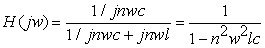(1)

In the formula, w is the fundamental angular frequency, n is the harmonic order, set w0 is the cut-off frequency, then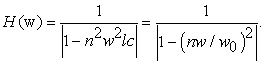(2)

Therefore, the smaller the cutoff frequency, the better the filtering effect. But if capacitance and inductance is too large, it will also affect the compensation effect. So take L=0.5mH and C=2μF.

3.2. Selection of Transformer Parameters

The compensation voltage output by the inverter side is mainly compensated by a transformer connected in series to the main circuit. The transformation ratio of the transformer depends on the DC bus voltage and the compensation interval.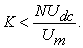(3)

In the formula, set the transformation ratio to K:1, the modulation ratio of the output maximum compensation voltage is N, the DC side voltage is Udc, and the grid voltage amplitude is Um.

Suppose the grid voltage amplitude is 380V. The DC voltage is 800V. The maximum compensation voltage modulation ratio is 1, and K<2.1 can be obtained. Here K=1, which means that the transformer here mainly plays the role of inputting the compensation voltage into the grid, and the role of transformation is not great.

3.3. Control Circuit Design

The voltage that needs to be compensated subtracts the voltage that is actually compensated, and then the amount of current that needs to be compensated is controlled by PI. The control equation is: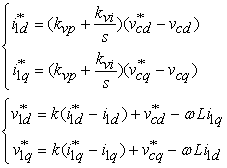(4)

It can be obtained that the control effect is best when kvp=1.2 and kvi=0.08 under PI control, and the compensated waveform is the most stable. Also due to the coupling relationship between the d and q axes, assuming a certain loss, when the loss inductance L=0.51mH, the d-axis current multiplied by the inductance acts on the q-axis, and the q-axis current multiplied by the inductance acts on the d-axis. At this time, the compensation effect is the best, and can represent the coupling relationship between the dq axis. The control principle diagram is shown in Figure 3.

• Figure 3. Control principle diagram
3.4. DC Energy Storage Unit Design

Capacitors are used as energy storage components to ensure the stability of the DC side voltage. The stability of the DC side voltage is the prerequisite for stable compensation voltage. In this paper, capacitors are used as energy storage components, which are easy to control and cost-effective. Therefore, the choice of capacitor size determines the stability of the compensation voltage. The energy stored in the capacitor is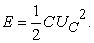(5)

In the formula, UC is the voltage across the capacitor. The energy storage of the capacitor must not only ensure the normal operation of the converter, but also compensate the active power of the load. According to the debugging, it is found that when the capacitor size is 1μF and the resistance size is 100Ω, the capacitor can maintain a voltage drop for a certain period of time, which is able to realize complete compensation and stable output of compensation voltage.

### 4. Simulation Results

The dynamic voltage compensator matlab simulates the power failure and three-phase short-circuit failure. The inverter system is through the SPWM system. That is, the difference between the modulating wave and the carrier wave is the PWM pulse, which is input into the three-phase bridge inverter system. The detection system uses an improved synchronous coordinate transformation detection method. The angle of the d-axis and the angle of the a-axis are arbitrarily selected. That is, the fundamental positive sequence voltage can be calculated from the direct current in the dq coordinate system. Convert all the variables to the dq coordinate system, and then pass the control module. The control module considers the influence of dq coupling, and obtains the compensation amount by comparing the reference voltage with the voltage after the sag is detected. Set up a branch to speed up compensation. By controlling the output current of the inverter system through the PI system based on the difference between the theoretical compensation voltage and the actual compensation voltage, the compensation process can be accelerated and the output more stable.

The power supply voltage adopts a three-phase programmable power supply, the voltage effective value is 220V, the phase is 30°, the frequency is 50HZ, the simulation time is 1s, the power failure time is 0.2s to 0.26s, the voltage drop is 50%, and the three-phase short circuit failure time is 0.4s, and return to normal at 0.45s.

4.1. Voltage Compensation

As shown in Figure 4 and Figure 5, the dynamic voltage restorer compensates the load voltage. When the power fails, the voltage drops to 50% of the normal voltage. The compensation effect is better, and the harmonics generated are less. When a three-phase short circuit occurs, some harmonics will be generated. After many debugging, it is found that the larger the inductance, the better the filtering effect. So finally a filter element with a resistance of 0.1 ohm and an inductance of 10000 μH is added to make the compensation effect better.

4.2. Power Compensation
4.2.1. Active Power Compensation

It can be seen from Figure 6 and Figure 7 that When power failure and three-phase short circuit occur, both active and reactive power will fluctuate. Therefore, In addition to voltage compensation, DVR also compensates for active power and reactive power.

• Figure 4. Voltage before compensation
• Figure 5. Voltage after compensation
• Figure 6. Output active power waveform
• Figure 7. Output reactive power waveform

### 5. Conclusion

This paper studies the working principle and basic structure of the dynamic voltage restorer, and introduces the corresponding function of each part of the structure. The parameter selection method is discussed for each component, and a voltage and current double closed-loop control strategy is proposed. And in the simulation, the power failure and three-phase short-circuit failure are simulated, which verifies the effectiveness of the control strategy. All in all , it can compensate the voltage in time, and also compensate the active power and reactive power. Propose a new solution for such problems in the future.

### References

  Duan Qing, Sheng Wanxing, Guo Qi, Sha Guanglin, Sun Yong, Zhang Li. Research on optimal output and energy self-recovery strategy of cascaded H-bridge dynamic voltage restorer[J]. Power System Technology, 2020, 44(03): 1079-1089. In article  Tu Chunming, Zhang Li, Guo Qi, Jiang Fei, Sun Yong. Research on Maximum Level Output Characteristics of Dynamic Voltage Restorer Based on HPWM Modulation[J]. Power System Technology. 2019(08). In article  Gee A M, Robinson F, Yuan W. A Superconducting Magnetic Energy Storage-Emulator/Battery Supported Dynamic Voltage Restorer. IEEE Transactions on Energy Conversion. 2017. In article View Article  Zeng Jinhui, Song Zhijie, Lan Zheng, He Jiaqi, Zhao Yongchao, Luo Jiao. Dynamic voltage restorer based on comprehensive energy optimal compensation [J/OL]. Electrical measurement and instrumentation: 1-8 [2020-08-14]. In article  A novel DVR-ESS-embedded wind-energy conversion system. Chen X, Yan L, Zhou X, et al. IEEE Transactions on Sustainable Energy. 2018. In article  Liu Zhenyu. Research on dynamic voltage restorer based on neuron adaptive control [J]. Electric Machines & Control Application, 2020, 47(07): 53-57. In article  Du Li. Research on voltage sag detection and compensation method of dynamic voltage restorer [D]. Jiangsu University, 2017. In article  Yang Hao, Shen Yechao. DVR dynamic performance research based on dual-loop vector decoupling control strategy[J]. Journal of Jianghan University (Natural Science Edition), 2019, 47(05): 416-423. In article  Tu Chunming, Zhang Li, Guo Qi, Jiang Fei, Sun Yong. Research on the maximum level output characteristics of dynamic voltage restorer based on HPWM modulation[J]. Power System Technology, 2019, 43(08): 2942-2951. In article  Gai Kuo, An Quntao, Sun Li. Harmonic compensation strategy of dynamic voltage restorer based on multiple proportional resonance[J]. Power Automation Equipment, 2018, 38(01): 156-161. In article  Sha Ou, Sun Yukun, Zhang Liang, Chen Hui. Research on Dynamic Voltage Restorer Based on Super Capacitor Energy Storage[J]. Electrical Measurement and Instrumentation, 2014, 51(13): 98-102+111. In article  Tu Chunming, Sun Yong, Guo Qi, Jiang Fei, Zhang Li. Minimum energy flexible switching control strategy suitable for dynamic voltage restorer[J]. Journal of Electrical Engineering Technology. 2019(14). In article  Li Zhengming, Li Wenwen, Zhang Guosong, Liu Liang. A DVR optimal compensation strategy for compensating grid voltage sag[J]. Power system protection and control. 2016(17). In article View Article  Chen Qi, Xiong Lianggen, Liu Shujun, Yao Liang. Research on dynamic voltage restorer based on double dq conversion software phase lock[J]. Power system protection and control. 2015(04). In article  CASTILLA M,MIRET J, CAMACHO A, et al. Voltage support control strategies for static synchronous compensators under unbalanced voltage sags. IEEE Transactions on Industrial Electronics. 2014. In article View Article  Wang Shiwei, Huang Ke, Xu Xingtao. Non-current inner loop control strategy in dynamic voltage restorer[J]. Automation of Electric Power Systems. 2014(18). In article  Chen Ye. Research on DVR control technology based on multi-phase interleaved parallel Buck circuit[D]. Harbin Institute of Technology, 2019. In article  Zhang Huijie, Hao Ruilin. Research on nonlinear control of dynamic voltage restorer in power grid[J]. Electronic Testing. 2019(Z1). In article  Yao Gang, Ji Feipeng, Yin Zhizhu, Zhou Lidan. Topology analysis and control of a DC dynamic voltage restorer[J]. Power Electronics Technology. 2017(11). In article  Mohammad Farhadi-Kangarlu, Ebrahim Babaei, Frede Blaabjerg. A comprehensive review of dynamic voltage restorers[J]. International Journal of Electrical Power and Ene . 2017. In article View Article

Published with license by Science and Education Publishing, Copyright © 2020 Yuqi Pang, Xiaotian Xu, Xunyu Liu and Xinyuan ZhangThis work is licensed under a Creative Commons Attribution 4.0 International License. To view a copy of this license, visit http://creativecommons.org/licenses/by/4.0/